This section provides a brief overview of a complementary problem to motion planning. Suppose that an algorithm in this chapter returns a feasible action trajectory. How can the solution be improved? Trajectory optimization refers to the problem of perturbing the trajectory while satisfying all constraints so that its quality can be improved. For example, it may be desirable to shorten a trajectory computed by an RRT, to remove some of the arbitrary changes in actions due to randomization. Trajectory optimization is considered complementary to motion planning because it usually requires an initial guess, which could be provided by a planning algorithm. Trajectory optimization can be considered as a kind of BVP, but one that improves an initial guess, as opposed to determining trajectories from scratch.

The optimization issue also exists for paths computed by sampling-based algorithms for the Piano Mover's Problem; however, without differential constraints, it is much simpler to shorten paths. The plan and transform method of Section 14.6.2 can be applied, and the LPM just connects pairs of configurations along the shortest path in. In the presence of differential constraints, the BVP must be faced.

In the most general setting, it is very difficult to improve trajectories. There are numerous methods from optimization literature; see [98,151,664] for overviews. The purpose of this section is to encourage further study by briefly mentioning the various kinds of methods that have been developed, instead of explaining them in detail. The methods fall under the area of nonlinear programming (NLP) (or nonlinear optimization), as opposed to linear programming, which was used to find randomized security strategies in Section 9.3. The optimization actually occurs in a space of possible trajectories, each of which is a function of time. Therefore, the calculus of variations, which was used in Section 13.4.1, becomes relevant to characterize extrema. The functional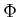from that setting becomes the cost functional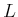in the current setting. The system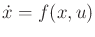forms an additional set of constraints that must be satisfied, butcan be selected in the optimization.

To enable numerical computation methods, a family of trajectories is specified in terms of a parameter space. The optimization can then be viewed as an incremental search in the parameter space while satisfying all constraints. The direction of motion in each step is determined by computing the gradient of a cost functional with respect to the parameters while constrained to move in a direction tangent to the constraints. Hence, much of nonlinear programming can be considered as an application of Newton's method or gradient descent. As in standard optimization, second-order derivatives of the cost functional can be used to indicate when the search should terminate. The numerical issues associated with these methods are quite involved; several NLP software packages, such as the NAG Fortran Library or packages within Matlab, are available.

Nonlinear optimal control theory can be considered as a variant of NLP. The dynamic programming recurrence becomes a differential equation in the continuous-time setting, and Hamilton's equations (13.198) generalize to Pontryagin's minimum principle. These are covered in Section 15.2. The extra variables that arise in the minimum principle can be considered as Lagrange multipliers of a constrained optimization, in whichis the constraint. The differential equations arising from dynamic programming or the minimum principle are difficult to solve analytically; therefore, in most cases, numerical techniques are used. The case of numerical dynamic programming was covered in Section 14.5.

Shooting methods constitute the simplest family of trajectory optimization methods. As a simple example, suppose that an action trajectory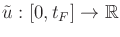has been computed of the form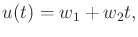(14.47)

in which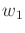and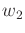are some fixed parameters. Consider perturbingandby some small amount and applying the integration in (14.1). If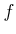satisfies Lipschitz conditions, then a small perturbation should produce a small change in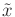. The resulting new trajectory can be evaluated by a cost functional to determine whether it is an improvement. It might, for example, have lower maximum curvature. Rather than picking a perturbation at random, the gradient of the cost functional with respect to the parameters can be computed. A small step in the parameter space along the negative gradient direction should reduce the cost. It is very likely, however, that perturbingandwill move the final state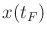. Usually, a termination condition, such as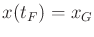, must be enforced as a constraint in the optimization. This removes degrees of freedom from the optimization; therefore, more trajectory parameters are often needed.

Suppose more generally that a motion planning algorithm computes an action sequence based on the discrete-time model. Each action in the sequence remains constant for duration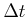. The time duration of each action can instead be defined as a parameter to be perturbed. Each action variableover each interval could also be perturbed using by (14.47) with the initial condition that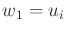and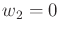. The dimension of the search has increased, but there are more degrees of freedom. In some formulations, the parameters may appear as implicit constraints; in this case, a BVP must be solved in each iteration. The minimum principle is often applied in this case . More details on formulating and solving the trajectory optimization problem via shooting appear in .

Several difficulties are encountered when applying the shooting technique to trajectory optimization among obstacles. Each perturbation requires integration and collision-checking. For problems involving vehicles, the integrations can sometimes be avoided by exploiting symmetries . For example, a path for the Dubins car can be perturbed by changing a steering angle over a short amount of time, and the rest of the trajectory can simply be transformed using a matrix of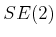. A critical problem is that following the negative gradient may suggest shortening the path in a way that causes collision. The problem can be alleviated by breaking the trajectory into segments, as in the plan-and-transform approach; however, this yields more optimizations. Another possible solution is to invent a penalty function for the obstacles; however, this is difficult due to local minima problems and the lack of representing the precise boundary of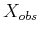.

Another difficulty with shooting is that a small change in the action near the starting time may lead to great changes in the states at later times. One way to alleviate this problem is by multiple shooting (as opposed to single shooting, which has been described so far). In this case, the trajectory is initially broken into segments. These could correspond to the time boundaries imposed by a sequence of motion primitives. In this case, imagine perturbing each motion primitive separately. Extra constraints are needed in this case to indicate that all of the trajectory pieces must remain connected. The multiple shooting method can be generalized to a family of methods called transcription or collocation (see  for references). These methods again split the trajectory into segments, but each connection constraint relates more points along the trajectory than just the segment endpoints. One version of transcription uses implicit constraints, which require using another BVP solver, and another version uses parametric constraints, which dramatically increases the dimension of the search. The latter case is still useful in practice by employing fast, sparse-matrix computation methods.

One of the main difficulties with trajectory optimization methods is that they can become stuck in a local minimum in the space of trajectories. This means that their behavior depends strongly on the initial guess. It is generally impossible for them to find a trajectory that is not homotopic to the initial trajectory. They cannot recover from an initial guess in a bad homotopy class. Ifis complicated, then this issue becomes increasingly important. In many cases, variational techniques might not even find an optimal solution within a single homotopy class. Multiple local minima may exist if the closure of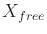contains positive curvature. If it does not, the space is called nonpositively curved (NPC) or CAT(0), which is a property that can be derived directly from the metric on. For these spaces, the locally optimal trajectory with respect to the metric is always the best within its homotopy class.

Subsections
Steven M LaValle 2020-08-14